CIS 5511. Programming Techniques

Greedy Algorithms

### 1. The idea

Greedy algorithm is another method often used for optimization problems. Such an algorithm always makes the locally optimal choice (i.e., the next step that looks the best at the moment), in the hope that it will lead to a globally optimal solution.

Unlike dynamic programming, a greedy algorithm may fail to find the optimal solution, though it usually finds a pretty good one, and runs much faster than an algorithm using dynamic programming.

### 2. Example: Activity Selection

Problem: for a given set of activities A1 ... An, each with its start and finish time for using a resource exclusively, find a schedule that maximize the usage of the resource, defined by the number of compatible activities.

A greedy solution: recursively find the activity with the earliest finish time, and add it into the schedule.

In the following algorithm, array s and f represents the start and finish time of the activities, and they are sorted in monotonically increasingly order of finish time: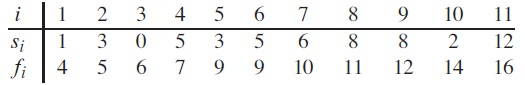Beside the two arrays, the algorithm also takes indexes k and n as input, indicate the range of activities to be considered. Initially, it is called as Recursively-Activity-Selector(s, f, 0, n).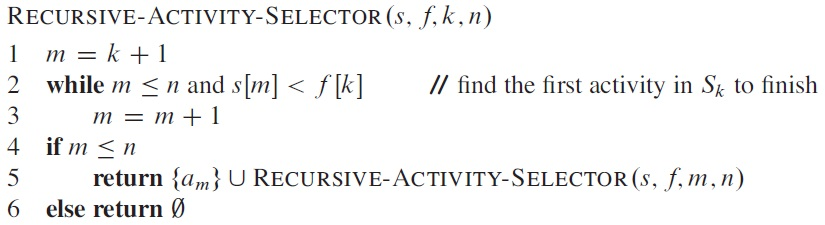The recursive algorithm can be converted into a more efficient iterative algorithm: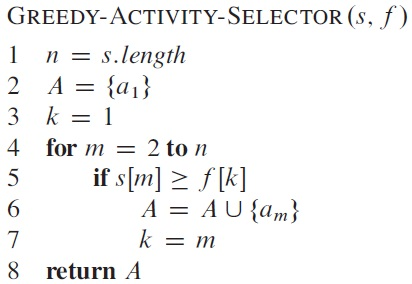Both algorithms take Θ(n) time.

### 3. Example: Huffman coding

Huffman coding is an efficient way to compress data for communication and storage. The idea is to use variable-length code for data units, with higher-frequent ones with shorter codes. There is no ambiguity as long as no codeword is also a prefix of some other codeword.

In the following example, the variable-length codeword has an average of 2.24 bits: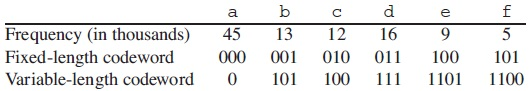Assuming binary prefix coding, a solution can be represented by a binary tree, with each leaf corresponds to an unit, and its path from root represents the code for the unit. An optimal code is a binary tree with the minimum expected path length.

In the following algorithm, C contains a set of units, and Q is a priority queue containing binary trees prioritied by the frequency of their roots.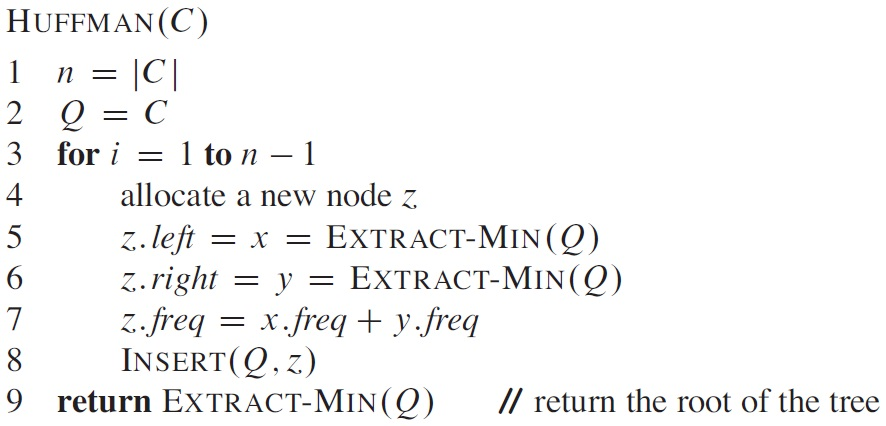Procedure HUFFMAN produces an optimal prefix code. Example: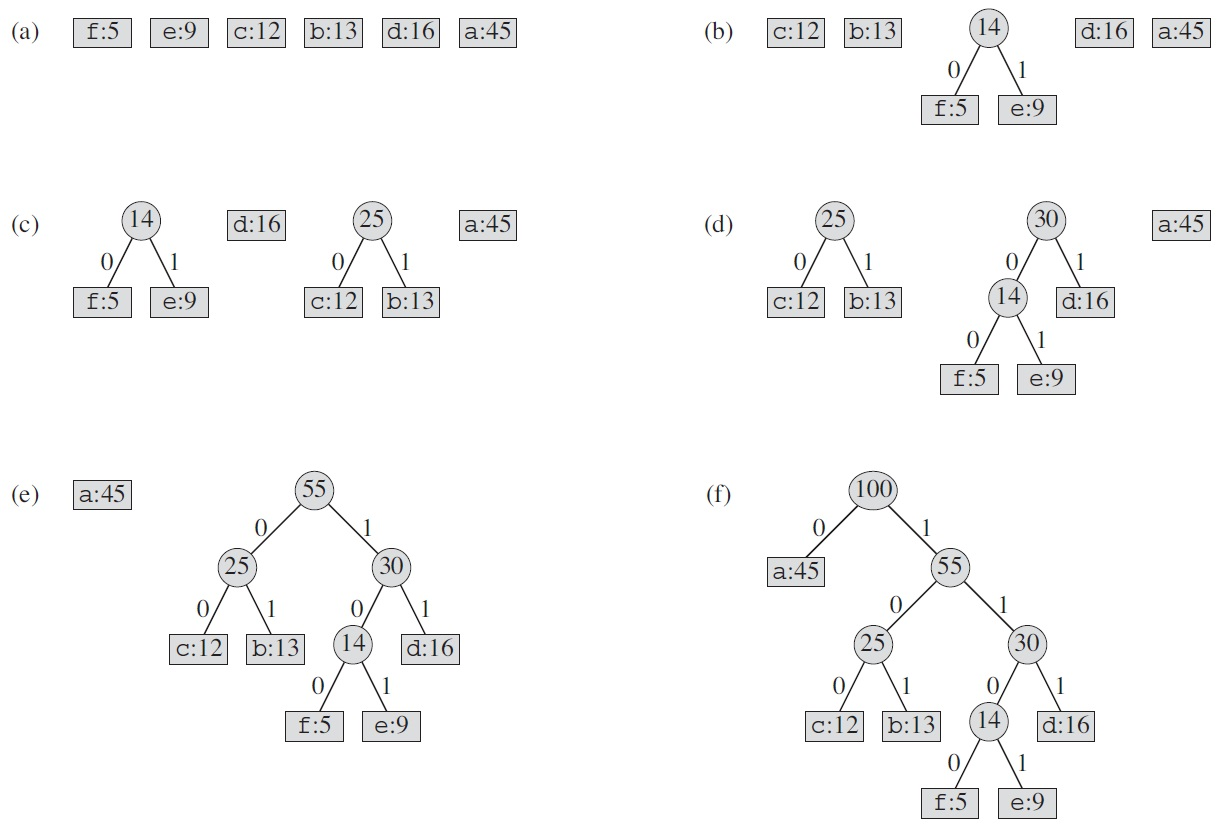### 4. Related techniques

Hill Climbing: When an optimization problem can be represented as searching for the maximum value of a function in a multidimensional space, "hill climbing" is a greedy algorithm which attempts to find a better solution by making an incremental change to the current solution. [Reference 1 Reference 2]

Best-First Search: If the next step is selected at the neighborhood of all explored points, "hill climbing" becomes "best-first search". As the selection is based on a heuristic function, it is also called heuristic search. [Reference 1 Reference 2]

Anytime Algorithm: Such an algorithm optimizes the quality of its solution over time, and can stop at anytime with a best-so-far solution. It is greedy in the sense that each solution is usually produced from local consideration only. An anytime algorithm no longer has a determined solution and running time for a given problem instance. [Reference 1 Reference 2]